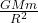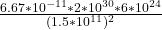## The mass of the Sun is 2multiply1030 kg, and the mass of the Earth is 6multiply1024 kg. The distance from the Sun to the Earth is 1.5multipl

Question

The mass of the Sun is 2multiply1030 kg, and the mass of the Earth is 6multiply1024 kg. The distance from the Sun to the Earth is 1.5multiply1011 m. (a) Calculate the magnitude of the gravitational force exerted by the Sun on the Earth. N (b) Calculate the magnitude of the gravitational force exerted by the Earth on the Sun.

in progress 0
2 months 2021-07-25T16:45:54+00:00 1 Answers 7 views 0

a) 3.56 x 10^22 N

b) 3.56 x 10^22 N

Explanation:

Mass of the sun M = 2 x 10^30 kg

mass of the Earth m = 6 x 10^24 kg

Distance between the sun and the Earth R = 1.5 x 10^11 m

From Newton’s law,

F =where F is the gravitational force between the sun and the Earth

G is the gravitational constant = 6.67 × 10^-11 m^3 kg^-1 s^-2

m is the mass of the Earth

M is the mass of the sun

R is the distance between the sun and the Earth.

Substituting values, we have

F == 3.56 x 10^22 N

A) The force exerted by the sun on the Earth is equal to the force exerted by the Earth on the Sun also, and the force is equal to 3.56 x 10^22 N

b) The force exerted by the Earth on the Sun = 3.56 x 10^22 N